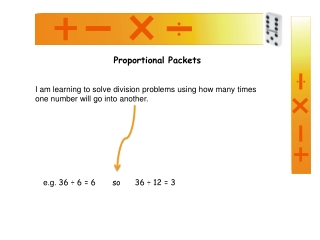DownloadDownload PresentationProportional Packets

# Proportional Packets

Download Presentation## Proportional Packets

- - - - - - - - - - - - - - - - - - - - - - - - - - - E N D - - - - - - - - - - - - - - - - - - - - - - - - - - -
##### Presentation Transcript

1. Proportional Packets I am learning to solve division problems using how many times one number will go into another. e.g. 36 ÷ 6 = 6 so 36 ÷ 12 = 3

2. There are twenty counters below which must be split into 4 groups. How many counters will there be in each group? 20 ÷ 4 = 5 you may have worked this out using your multiplication facts e.g. 4 X what = 20. The answer is 5 so there must be 5 in each group. Think about how many counters you would have if you only had two groups (half as many groups). So if we have half as many groups then we must put twice as many counters in each group. 20 ÷ 4 = 5 x 2 (twice as many in each group) ÷ 2 (half as many groups) 20 ÷ 2 = 10

3. Let’s imagine we still have 30 counters to split into 5 groups. 30 ÷ 5 = 6 What will happen if we have twice as many groups? How many counters will we need to put in each group? So if we have twice as many groups then we only need half as many counters in each group. 30 ÷ 5 = 6 x 2 (twice as many in each group) ÷ 2 (half as many groups) 30 ÷ 10 = 3

4. There are 27 counters below which are split into 3 groups. 27 ÷ 3 = 9 What will happen if we have three times as many groups? How many counters will we need to put in each group? So if we have three times as many groups then we put three times less in each group. 27 ÷ 3 = 9 x 3 (three times as many groups) ÷ 3 (three times less in each group) 27 ÷ 9 = 3

5. The questions on the left have a buddy question to the right where, doubling and halving or trebling and thirding has been used. Which questions do you think go together. • 18 ÷ 3 = ? • 48 ÷ 4 = ? • 3) 120 ÷ 5 = ? • 4) 60 ÷ 12 = ? 6 60 ÷ 6 = 10 Click again when you have worked out which questions go together. 12 120 ÷ 10 = 12 24 18 ÷ 9 = 2 48 ÷ 8 = 6 5

6. Now try these questions on your own. Click again when you have worked out all your answers. 64 ÷ 8 = 8 So 64 ÷ 4 = 16 54 ÷ 6 = 9 So 54 ÷ 3 = 18 45 ÷ 9 = 5 So 45 ÷ 3 = 15 180 ÷ 10 = 18 So 180 ÷ 5 = 36 72 ÷ 8 = 9 So 72 ÷ 4 = 18 1) 64 ÷ 4 = ? 2) 54 ÷ 3 = ? 3) 45 ÷ 3 = ? 4) 180 ÷ 5 = ? 5) 72 ÷ 4 = ? Need some more practise? Try Pg35 & 36 of Teacher Tools Multiplication and Division Book Numeracy resources